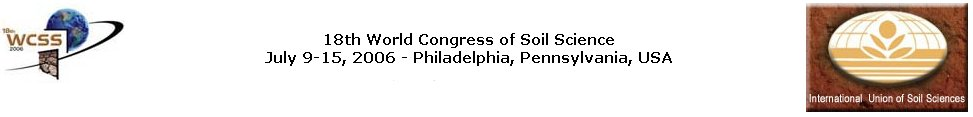### Application of a General Relationship between Soil Particle Density and Organic Matter to Mollisols of Santa Fe (Argentina).

Miguel A. Pilatti, Pablo J. Ghiberto, and Silvia Imhoff. Facultad de Ciencias Agrarias, Universidad Nacional del Litoral, 2805 Kreder Street, Esperanza, Argentina

The soil particle density (dp) is useful to calculate total porosity and particle size distribution of the soils. dp synthesizes the contribution of diverse minerals and organic components present in the studied material. For most of the mineral soils dp oscillates between 2.6 and 2.75 Mg m-3 due to the predominance of quartz, feldspar, micas and clay minerals. Organic matter possesses a density that varies between 0.9 and 1.3 Mg m-3. The phytoliths (2.21 Mg m-3) presence, common in the light fraction of silt of A horizons, can modify the mean value of dp of the Pampas soils in Argentina. The Pampas Loess is the parent material of a great proportion of soils in Santa Fe, where silicates and volcanic glasses prevail. For this reason, dp depends basically on these minerals and on the organic matter content. The knowledge of dp for Mollisols is very important because they are the most productive soils, occupying 57% of the province. Pedotransfer functions, generally lineal, have been elaborated to estimate dp for several soils. However, this information is not available for soils of Santa Fe. A general relationship between dp, mineral particles density and organic matter is proposed. It is a physically based deduction that, besides being useful as a pedotransfer function, allows knowing separately the organic matter density and the minerals density of the studied soil. In addition, it is also possible to distinguish if the fractions sand, silt and clay have different density values. The general equation is:

100/dp = 100/di + C ((di - dp)/(di do))

Where: dp is particle density, di and do are the mineral and organic particles densities respectively (g cm-3), and C is the percentage of organic matter.

The linear form of the previous equation is: Y = a + bX

Where:

Y = 100/dp; a = 100/di; and b = (di - do)/(di do)

Consequently, the mineral density is: di = 100/a

The organic matter density is: do = 100/(100 b + a)

Similarly, the General Equation can be expanded to know the density of the fractions clay (cl), silt (si), and sand (sa):

1/dp = cl/dcl + si/dsi + sa/dsa + C/do

The previous equation can be expressed, using multiple regression, as shown:

Y = a X1 + b X2 + c X3 + d X4

Where: Y = 100/dp, and X1, X2, X3, and X4 are clay, silt, sand, and organic matter contents (g 100g-1 of dry soil).

The density of each fraction is defined as:

dcl = 1/a; dsi = 1/b; dsa = 1/c; do = 1/d

The objectives of this work are: i) to evaluate if the proposed general equation is valid for Mollisols of the Santa Fe State (Argentina), and ii) to deduce the mineral and organic matter densities. Disturbed soil samples (n=50) were used to determine dp (by Pycnometer method) and organic matter content (OM) (Walkley and Black) of A, B, C, and transitional horizons of Mollisols located between 28 and 34ş S Latitude, and 59 and 62ş W Longitude. The results were adjusted to a lineal regression model. The coefficient of determination was calculated. Figure 1 shows the dp behavior with the increase in the organic matter content of the studied Mollisols. It was deduced that the minerals density is 2.656 g cm-3, and the organic matter density is 1.076 g cm-3. However, it has to be noted that the fit of the data is not very high, especially when organic matter values are between 1 and 2%. This fact can be attributed at least to two reasons: 1) the technique used to determine organic matter content doesn't have the same recovery capacity for all horizons, being smaller in the B horizons; 2) it is possible that the density of the sand, silt and clay fractions be different in the Mollisols of Santa Fe. Future researches need to elucidate these aspects